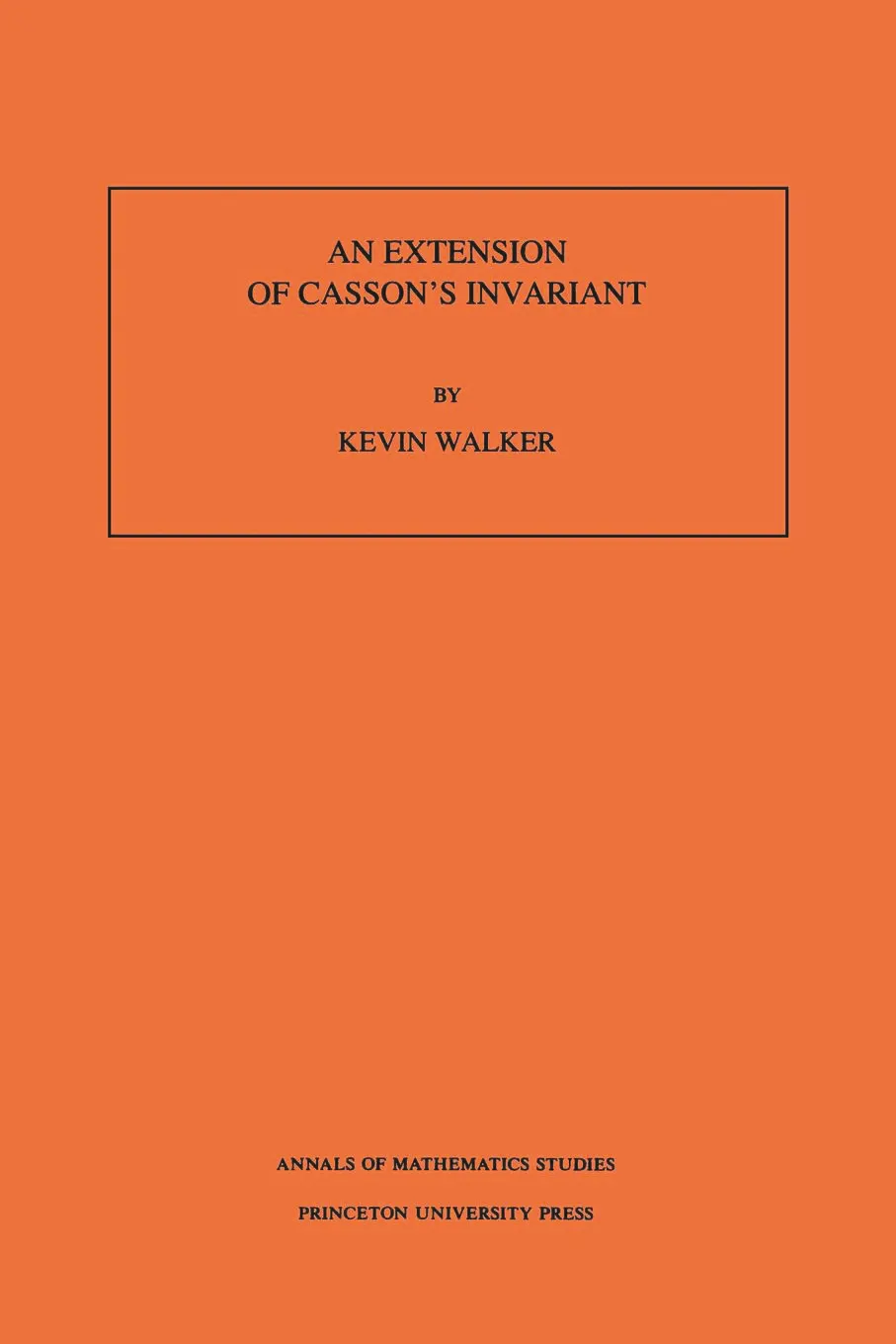#### An Extension of Casson's Invariant. (AM-126), Volume 126

Kevin Walker

##### ebooks

Many of our ebooks are available for purchase from these online vendors:

Many of our ebooks are available through library electronic resources including these platforms:

# An Extension of Casson's Invariant. (AM-126), Volume 126## Paperback

Price:
\$67.50 / £54.00
ISBN:
Published:
Mar 23, 1992
1992
Pages:
150
Size:
6 x 9.25 in.

This book describes an invariant, l, of oriented rational homology 3-spheres which is a generalization of work of Andrew Casson in the integer homology sphere case. Let R(X) denote the space of conjugacy classes of representations of p(X) into SU(2). Let (W,W,F) be a Heegaard splitting of a rational homology sphere M. Then l(M) is declared to be an appropriately defined intersection number of R(W) and R(W) inside R(F). The definition of this intersection number is a delicate task, as the spaces involved have singularities.

A formula describing how l transforms under Dehn surgery is proved. The formula involves Alexander polynomials and Dedekind sums, and can be used to give a rather elementary proof of the existence of l. It is also shown that when M is a Z-homology sphere, l(M) determines the Rochlin invariant of M.